# Case Study: The Federalist Papers

Patrick O. Perry, NYU Stern School of Business

## Overview

We will replicate the analysis performed by Mosteller and Wallace (1963) to determine the authorhips of the 15 disputed Federalist Papers.

## Preliminaries

### Computing environment

We will use the following R packages. You can see the exact version numbers and the rest of my R session information at the end of this document.

``````library("dplyr")
library("jsonlite")
library("Matrix")
library("NLP")
library("openNLP")
library("SnowballC")
library("tm")
``````

We will also use some code for fitting negative binomial models.

``````source("nbinom.R") # 'nbinom.R' must be in the working directory
``````

To ensure consistent runs, we set the seed before performing any analysis:

``````set.seed(0)
``````

### Data

The raw text for the Federalist Papers is available from Project Gutenberg. I have processed the raw text to produce a newline-delimited JavaScript Object Notation (JSON) data file with one record for each of the 85 papers, named federalist.json.

We can read this file into R using the `stream_in` function from the `jsonlite` package.

``````fed <- jsonlite::stream_in(file("federalist.json"))
``````
``````
Found 85 lines...
``````

Each record has 6 fields.

``````names(fed)
``````
`````` "author"   "text"     "date"     "title"    "paper_id" "venue"
``````

The authorships reported by the Project Gutenenberg versions are as follow.

``````fed %>% group_by(author) %>% summarize(count = n())
``````
``````Source: local data frame [5 x 2]

author count
1             HAMILTON    51
4                  JAY     5
``````

The text of the paper is stored in the `"text"` field.

The Project Gutenberg version of the Federalist Papers attributes paper No. 58 to Madison, but Mosteller and Wallace consider this paper to have disputed authorship. We will follow Mosteller and Wallace in our subsequent analysis.

``````fed\$author[fed\$paper_id == 58] <- "HAMILTON OR MADISON"
``````

## Exploratory analysis

### Sentence length

First we break each document into sentences.

``````# use the 'openNLP' maximum entropy sentence annotator
ator <- openNLP::Maxent_Sent_Token_Annotator(language="en")

ntext <- nrow(fed)
sents <- vector("list", ntext)

for (i in seq_len(ntext)) {
# convert the text to a 'String' object to annotate it
s <- NLP::as.String(fed\$text[[i]])

# compute the sentence boundaries
spans <- NLP::annotate(s, ator)
nsent <- length(spans)
sents[[i]] <- as.character(s[spans])
}
``````

Next, we compute the lengths (in words) of the sentences.

``````# use the 'wordpunct' word tokenizer
scan <- NLP::wordpunct_tokenizer

sents_nword <- vector("list", length(sents))
for (i in seq_along(sents)) {
nsent <- length(sents[[i]])
nword <- vector("numeric", nsent)
for (j in seq_len(nsent)) {
# convert the sentence to a string object
s <- NLP::as.String(sents[[i]][[j]])

# tokenize the sentence into words
spans <- scan(s)

# determine the sentence lengths
nword[[j]] <- length(spans)
}
sents_nword[[i]] <- nword
}
``````

For convenience, we store the sentence data in a data frame.

``````sents <- data_frame(paper_id = rep(fed\$paper_id, sapply(sents, length)),
text = c(sents, recursive=TRUE),
nword = c(sents_nword, recursive=TRUE))
``````

We can see that both Hamilton and Madison have similar sentence length averages and standard deviations:

``````# compute the sentence lengths for each author
(sents %>% left_join(fed, by="paper_id")
%>% group_by(author)
%>% summarize(n(), mean(nword), sd(nword)))
``````
``````Source: local data frame [5 x 4]

author  n() mean(nword) sd(nword)
1             HAMILTON 3384    37.18174  22.34336
2 HAMILTON AND MADISON  215    30.29767  19.69047
3  HAMILTON OR MADISON  784    34.22194  21.46343
4                  JAY  220    42.62273  21.15173
``````

The distributions of the sentence lenghts are also nearly identical.

``````h_sent_lens <- (sents %>% left_join(fed, by="paper_id")
%>% filter(author == "HAMILTON"))\$nword
m_sent_lens <- (sents %>% left_join(fed, by="paper_id")

par(mfrow=c(1,2))
cols <- paste0(palette(), "44")

# First Plot
#par(mar=c(3, 4, 1, 0.5) + .1)
hist(m_sent_lens, freq=FALSE, col=cols, 40, main="",
xlab="Sentence Length")

# Second Plot
hist(log10(m_sent_lens), freq=FALSE, col=cols, 40, main="",
xlab=expression(Log("Sentence Length")))
``````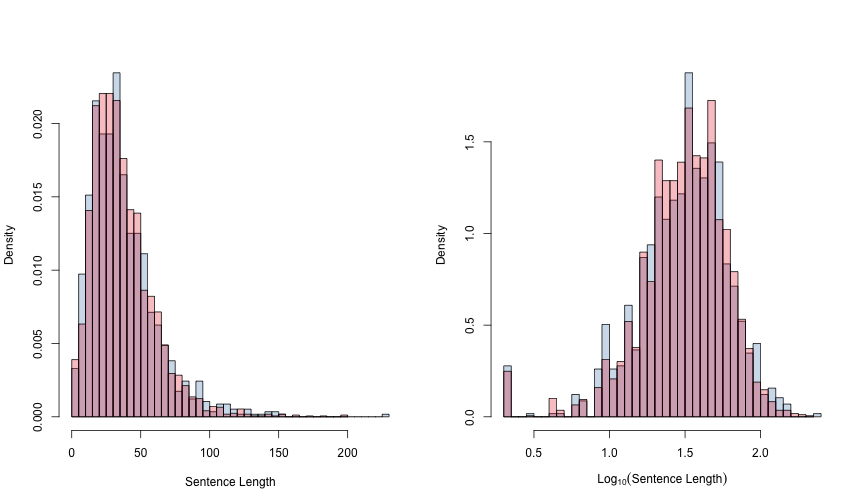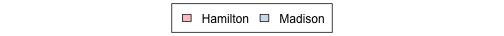Here are quantile plots for the two authors.

``````cols <- paste0(palette(), "AA")

par(mfrow=c(1,2))
hf <- (seq_along(h_sent_lens) - 0.5) / length(h_sent_lens)
hq <- sort(h_sent_lens)
mf <- (seq_along(m_sent_lens) - 0.5) / length(m_sent_lens)
mq <- sort(m_sent_lens)

# First Plot
plot(c(0, 1), range(hq, mq), type="n", xlab="Fraction",
ylab="Sentence Length")
lines(hf, hq, col=cols, lwd=3)
lines(mf, mq, col=cols, lwd=3)

# Second Plot
plot(c(0, 1), log10(range(hq, mq)), type="n", xlab="Fraction",
ylab=expression(Log("Sentence Length")))
lines(hf, log10(hq), col=cols, lwd=3)
lines(mf, log10(mq), col=cols, lwd=3)
``````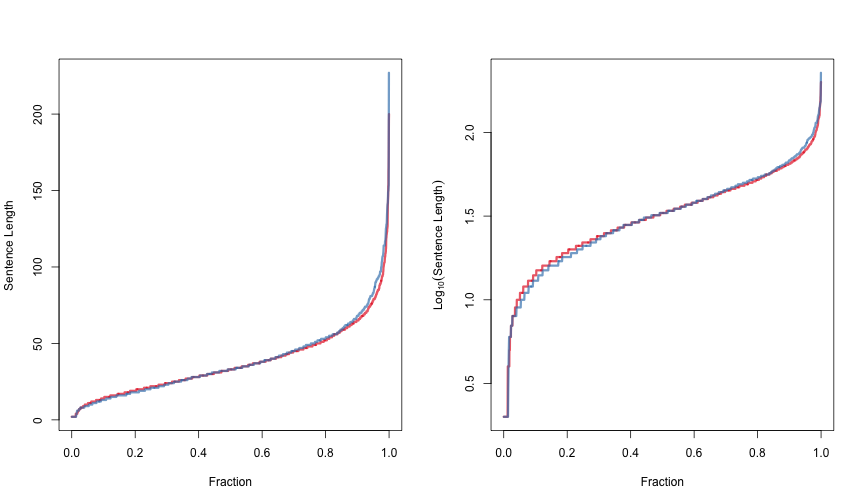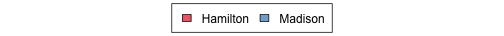In light of these similarities, it is unlikely that sentence length will be a good feature for discriminating between Hamilton and Madison.

### Word usage

To look at the word usages of the two authors, we form a “Document Term Matrix”, with rows corresponding to texts (papers) and columns corresponding to words. Entry (i,j) of the matrix will store the number of times that word j appears in text i.

``````stem <- function(words) {
words <- as.character(words)
long <- nchar(words) > 4
words[long] <- SnowballC::wordStem(words[long], language="porter")
words
}
corpus <- VCorpus(VectorSource(fed\$text))
control <- list(tolower = TRUE, removePunctuation = TRUE,
removeNumbers = TRUE, stemming = stem,
wordLengths=c(1, Inf))
dtm <- DocumentTermMatrix(corpus, control=control)
``````

The `DocumentTermMatrix` returns a sparse matrix in `simple_triplet_matrix` format, but for efficiency and consistency, I prefer to work sparse matrices in `sparseMatrix` format. The following code performs a conversion between these two types.

``````dtm <- sparseMatrix(dtm\$i, dtm\$j, x = dtm\$v, dim=dim(dtm),
dimnames=dimnames(dtm))
``````

To compare word usage behavior, we normalize by the length of the document, to get the rate of occurrence for each word.

``````rate <- dtm / rowSums(dtm)
``````

Here are side-by-side boxplots comparing the usage rates for six different words:

``````aut <- c("HAMILTON", "MADISON")
par(mfrow=c(2,3))
for (w in c("by", "from", "to", "war", "tax", "upon")) {
boxplot(1000 * rate[,w] ~ fed\$author, subset = fed\$author %in% aut,
ylab="Frequency / 1K Words", main=w)
}
``````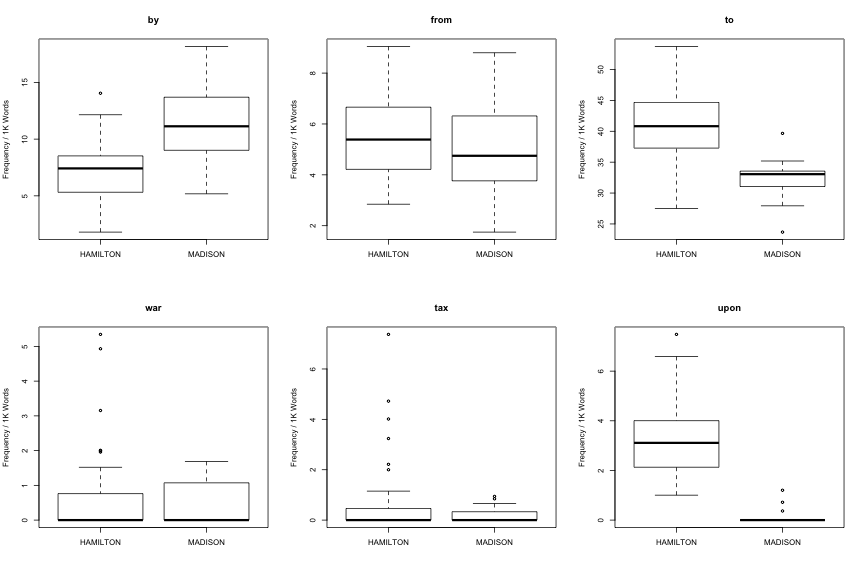We can see that for certain words (“by”, “to”, “upon”), usage rates very widely between the two authors. This suggests that these words can be used to discriminate between Hamilton and Madison. We can also see that for other words (“war”, “tax”), it is likely that most of the variability in usage is due to topic, not to author. We should avoid using these context-dependent words when determining paper authorship.

## Modeling word occurrence

### Purpose

We first need a probabilistic model for word occurrences in the texts. Mosteller and Wallace suggest using either a Poisson or Negative Binomial model.

### Fitting

The following code fits negative binomial models for each author and word.

``````author <- c("HAMILTON", "MADISON")
word <- colnames(dtm)

usage <-
do(data_frame(author) %>% group_by(author), { # for each author:
# extract the texts written by the author
x <- dtm[fed\$author == .\$author,]

# compute text lenghts
n <- rowSums(x)
offset <- log(n)

do(data_frame(word) %>% group_by(word), { # for each word:
# fit a negative binomial model for the word
y <- x[,.\$word]
fit <- nbinom_fit(y, n)

# compute the deviance for heterogeneity = 1
dev1 <- nbinom_pdev(y, offset, 0)
fit\$hetero1_deviance <- dev1

# return the results as a data frame (required by the 'do' command)
as.data.frame(fit)
}) %>% ungroup()
}) %>% ungroup()

# compute the chi squared statistics for H0 : hetero = 1 vs. H1: hetero < 1
usage <-
usage %>% mutate(chisq_hetero = ifelse(heterogeneity > 1,
deviance - hetero1_deviance,
hetero1_deviance - deviance),
pval_hetero = 1 - pchisq(chisq_hetero, df=1))
``````

### Diagnostic checks

To check the validity of the word occurrence models, we first segment the texts into blocks of about 200 words.

``````block_size <- 200
paper_id <- integer()
block_text <- list()
nblock <- 0

for (i in seq_len(nrow(fed))) {
# convert the text to a 'String' object to annote it
s <- NLP::as.String(fed\$text[[i]])

# find the word boundaries
spans <- NLP::wordpunct_tokenizer(s)
nword <- length(spans)

# form blocks of 'block_size' words
end <- 0
while (end < nword) {
# find the block boundaries
start <- end + 1
end <- min(start + block_size, nword)
block_span <- Span(spans[start]\$start, spans[end]\$end)

# store the block in the 'block_text' array
nblock <- nblock + 1
block_text[[nblock]] <- as.character(s[block_span])
paper_id[[nblock]] <- i
}
}

block <- data_frame(block_id = seq_len(nblock), paper_id = paper_id,
text = block_text)
dtm_block <- DocumentTermMatrix(VCorpus(VectorSource(block\$text)),
control=control)
dtm_block <- sparseMatrix(dtm_block\$i, dtm_block\$j, x = dtm_block\$v,
dim=dim(dtm_block), dimnames=dimnames(dtm_block))
``````

Here are the observed and expected counts under Hamilton's Poisson and negative binomial models for a few selected words.

``````# investigate goodness of fit for Hamilton, for a few selected words

h <- (block %>% left_join(fed, by="paper_id")
%>% filter(author == "HAMILTON"))\$block_id
x <- dtm_block[h,]
n <- rowSums(x)

for (w in c("an", "any", "may", "upon", "his", "can", "offic", "senat",
"would")) {
y <- x[, w]

fit <- usage %>% filter(author == "HAMILTON" & word == w)

table <-
do(data_frame(k=0:6) %>% group_by(k),
data_frame(observed=sum(y == .\$k),
pois_expected=sum(dpois(.\$k, fit\$pois_rate * n)),
nbinom_expected=sum(dnbinom(.\$k, size=1/(fit\$heterogeneity),
mu = fit\$rate * n)))
) %>% ungroup()

cat("\nWord: '", w, "'\n", sep="")
cat("Rate/1K: ", 1000 * fit\$pois_rate, "\n", sep="")
cat("Log(Heterogeneity): ", log(fit\$heterogeneity), "\n", sep="")
print(table %>% mutate(pois_expected=round(pois_expected, 1),
nbinom_expected=round(nbinom_expected, 1)))
}
``````
``````
Word: 'an'
Rate/1K: 5.634551
Log(Heterogeneity): -4.102734
Source: local data frame [7 x 4]

k observed pois_expected nbinom_expected
1 0      255         252.4           252.8
2 1      233         233.8           231.9
3 2      116         116.4           116.1
4 3       34          39.1            39.9
5 4       11           9.9            10.5
6 5        5           2.0             2.3
7 6        0           0.3             0.4

Word: 'any'
Rate/1K: 3.233666
Log(Heterogeneity): -2.124951
Source: local data frame [7 x 4]

k observed pois_expected nbinom_expected
1 0      381         376.5           384.5
2 1      200         205.3           195.3
3 2       57          58.9            58.4
4 3       14          11.4            13.0
5 4        1           1.7             2.4
6 5        1           0.2             0.4
7 6        0           0.0             0.1

Word: 'may'
Rate/1K: 4.190476
Log(Heterogeneity): -2.363592
Source: local data frame [7 x 4]

k observed pois_expected nbinom_expected
1 0      355         320.7           328.0
2 1      182         224.5           215.2
3 2       76          83.4            81.8
4 3       30          20.9            22.7
5 4        7           3.9             5.1
6 5        3           0.6             1.0
7 6        1           0.1             0.2

Word: 'upon'
Rate/1K: 3.3134
Log(Heterogeneity): -46.22818
Source: local data frame [7 x 4]

k observed pois_expected nbinom_expected
1 0      385         371.5           371.5
2 1      191         207.4           207.4
3 2       60          61.0            61.0
4 3       12          12.1            12.1
5 4        4           1.8             1.8
6 5        1           0.2             0.2
7 6        1           0.0             0.0

Word: 'his'
Rate/1K: 2.117386
Log(Heterogeneity): 0.814175
Source: local data frame [7 x 4]

k observed pois_expected nbinom_expected
1 0      550         454.9           499.5
2 1       44         163.9            99.6
3 2       26          30.9            33.3
4 3       18           3.9            12.7
5 4        4           0.4             5.1
6 5        8           0.0             2.1
7 6        2           0.0             0.9

Word: 'can'
Rate/1K: 2.586932
Log(Heterogeneity): -0.9928786
Source: local data frame [7 x 4]

k observed pois_expected nbinom_expected
1 0      452         420.0           432.0
2 1      139         184.2           164.2
3 2       45          42.3            44.7
4 3       15           6.6            10.4
5 4        1           0.8             2.2
6 5        1           0.1             0.4
7 6        0           0.0             0.1

Word: 'offic'
Rate/1K: 1.204873
Log(Heterogeneity): 0.8373279
Source: local data frame [7 x 4]

k observed pois_expected nbinom_expected
1 0      555         531.6           550.8
2 1       71         109.7            77.4
3 2       19          11.8            18.6
4 3        9           0.8             5.1
5 4        0           0.0             1.5
6 5        0           0.0             0.4
7 6        0           0.0             0.1

Word: 'senat'
Rate/1K: 1.187154
Log(Heterogeneity): 1.889989
Source: local data frame [7 x 4]

k observed pois_expected nbinom_expected
1 0      583         533.2           570.5
2 1       33         108.4            50.7
3 2       24          11.5            17.6
4 3        6           0.8             7.6
5 4        6           0.0             3.7
6 5        1           0.0             1.8
7 6        1           0.0             1.0

Word: 'would'
Rate/1K: 8.230343
Log(Heterogeneity): -1.242876
Source: local data frame [7 x 4]

k observed pois_expected nbinom_expected
1 0      289         166.0           201.3
2 1      123         216.2           192.7
3 2       99         156.1           128.0
4 3       61          76.5            70.4
5 4       31          28.3            34.6
6 5       26           8.4            15.7
7 6       11           2.1             6.7
``````

For most of these examples, the fit looks reasonable. For some words, (“his”, “senat”, “would”), the negative binomial is a much better fit than the Poisson model.

Here is a more comprehensive goodness of fit evaluation. For each word, we care the expected counts with the observed counts, then compute a Pearson chi-squared goodness of fit statistic.

``````gof <-
do(data_frame(author) %>% group_by(author), {
# extract the blocks for the current author
a <- .\$author
i <- (block %>% left_join(fed, by="paper_id")
%>% filter(author == a))\$block_id
x <- dtm_block[i,]
n <- rowSums(x)
do(data_frame(word=colnames(x)) %>% group_by(word), {
# extract the observed block counts and the fit for the current word
w <- .\$word
y <- x[, w]
fit <- usage %>% filter(author == a & word == w)

if (nrow(fit) == 0) {
# if a word appears in the block, but not in the original
# corpus, skip it
chisq <- NA
df <- NA
pval <- NA
pois_chisq <- NA
pois_df <- NA
pois_pval <- NA
} else {
# otherwise, perform two chi squared goodness of fit tests, one
# for the negative binomial model, and one for the Poisson
# model

# fitted parameters:
mu <- fit\$rate * n
size <- 1/fit\$heterogeneity
pois_mu <- fit\$pois_rate * n

# In the loop below, we perform a chi squared goodness of
# fit test by comparing the observed numbers of 0's, 1's,
# etc, to the number observed. We bin the counts so that
# the expected value is at least 5 in each cell.

# keep track of the remaining tail mass
ntail <- length(y)

# start out with one bin
expected <- numeric(1)
pois_expected <- numeric(1)
observed <- numeric(1)
nbin <- 1

k <- 0
while (ntail >= 5) {
# compute the observed and expected number of seeing
# the value 'k'
o <- sum(y == k)
if (!is.finite(size)) {
e <- sum(dpois(k, mu))
} else {
e <- sum(dnbinom(k, size=size, mu=mu))
}
pe <- sum(dpois(k, pois_mu))

# add the counts to the last bin
observed[nbin] <- observed[nbin] + o
expected[nbin] <- expected[nbin] + e
pois_expected[nbin] <- pois_expected[nbin] + pe

# update the tail mass
ntail <- ntail - o

# create a new bin if the observed count is at least 5
if (observed[nbin] >= 5 && ntail >= 5) {
nbin <- nbin + 1
observed[nbin] <- 0
expected[nbin] <- 0
pois_expected[nbin] <- 0
}

# advance to the next value of 'k'
k <- k + 1
}
# assign the remaining mass to the last bin
o <- ntail
if (!is.finite(size)) {
e <- sum(1 - ppois(k - 1, mu))
} else {
e <- sum(1 - pnbinom(k - 1, size=size, mu=mu))
}
pe <- sum(1 - ppois(k - 1, pois_mu))

observed[nbin] <- observed[nbin] + o
expected[nbin] <- expected[nbin] + e
pois_expected[nbin] <- pois_expected[nbin] + pe

# compute the chi squared statistics; the degrees of freedom
# here are approximate (cf. Chernoff and Lehmann, 1954)
chisq <-  sum((observed - expected)^2 / expected)
df <- nbin - 1
pval <- ifelse(df <= 0, NA, 1 - pchisq(chisq, df))

pois_chisq <-  sum((observed - pois_expected)^2 / pois_expected)
pois_df <- nbin - 1
pois_pval <- ifelse(pois_df <= 0, NA,
1 - pchisq(pois_chisq, pois_df))
}
data_frame(chisq, df, pval, pois_chisq, pois_df, pois_pval)
}) %>% ungroup()
}) %>% ungroup()
``````

Here are histograms of the goodness of fit p-values for both models:

``````par(mfrow=c(1,2))
hist(gof\$pval, 50, main="Negative Binomial Fit")
hist(gof\$pois_pval, 50, main="Poisson Fit")
``````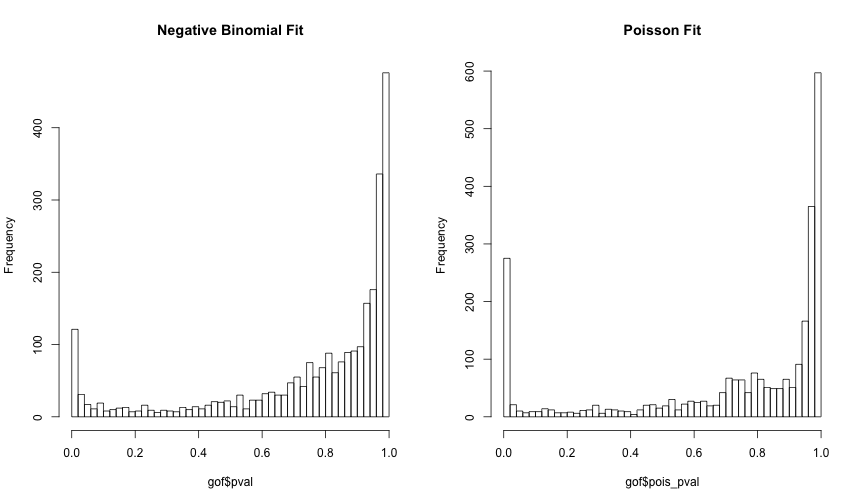Ideally, these histograms should be completely flat (the p-value should be uniformly distributed on `[0,1]` if the model fits). The skewed shape is due to the ad-hoc choice for the degrees of freedom. The skewness indicates that these goodness of fit tests are overly optimistic.

Here are the words with poor fits under the Poisson model, but reasonable fits under the negative binomial model:

``````print(n=20, gof %>% filter(pois_pval < .05 & pval > .05)
%>% arrange(pois_pval))
``````
``````Source: local data frame [136 x 8]

author      word      chisq df       pval pois_chisq pois_df    pois_pval
1  HAMILTON    execut  8.5211904  4 0.07424751  206.90203       4 0.000000e+00
2  HAMILTON      judg  4.3724102  3 0.22395727   93.43619       3 0.000000e+00
3  HAMILTON   militia  6.9069940  3 0.07492202  834.87150       3 0.000000e+00
4  HAMILTON     offic  1.0170848  3 0.79711804   92.30882       3 0.000000e+00
5  HAMILTON    presid  3.2622346  3 0.35293356  155.09658       3 0.000000e+00
6  HAMILTON     senat  9.1673253  4 0.05705054 1494.53569       4 0.000000e+00
7  HAMILTON       tax  5.4924767  3 0.13908929  417.01579       3 0.000000e+00
8   MADISON   appoint  6.5999922  3 0.08580138   85.31101       3 0.000000e+00
9   MADISON     power  9.9741411  6 0.12574523  125.43483       6 0.000000e+00
10  MADISON    govern 10.8405949  6 0.09342976   77.60647       6 1.110223e-14
11 HAMILTON    tribun  2.5701544  2 0.27662922   63.86013       2 1.354472e-14
12 HAMILTON     taxat  2.2495420  2 0.32472682   58.01428       2 2.525757e-13
13 HAMILTON     elect  4.7132897  2 0.09473755   57.19619       2 3.801404e-13
14 HAMILTON    suprem  4.9757649  2 0.08308572   52.52368       2 3.932077e-12
15 HAMILTON    treati  1.7339374  2 0.42022345   51.73080       2 5.845213e-12
16 HAMILTON      armi  1.5603919  2 0.45831620   50.81266       2 9.250600e-12
17  MADISON     their 10.7787258  5 0.05594806   54.49063       5 1.661481e-10
18 HAMILTON   impeach  0.1006048  2 0.95094180   43.75006       2 3.160788e-10
19 HAMILTON      vote  2.9034239  2 0.23416906   41.77317       2 8.493208e-10
20 HAMILTON constitut  7.4745913  4 0.11283494   47.24377       4 1.356647e-09
..      ...       ...        ... ..        ...        ...     ...          ...
``````

Here are the words with the worst goodness of fits under the negative binomial model:

``````print(n=20, gof %>% arrange(pval))
``````
``````Source: local data frame [10,434 x 8]

author  word     chisq df         pval pois_chisq pois_df    pois_pval
1  HAMILTON  will  91.93580  7 0.000000e+00  700.46452       7 0.000000e+00
2  HAMILTON would 100.61206  8 0.000000e+00  642.55956       8 0.000000e+00
3  HAMILTON    or  60.79139  6 3.107914e-11  108.37424       6 0.000000e+00
4  HAMILTON    we  54.55031  5 1.615189e-10  335.17728       5 0.000000e+00
5  HAMILTON   our  49.73851  4 4.094521e-10  517.43426       4 0.000000e+00
6  HAMILTON    as  54.68161  6 5.374943e-10   61.51171       6 2.217937e-11
7  HAMILTON  they  50.18425  5 1.270555e-09  101.23651       5 0.000000e+00
8  HAMILTON  jury  44.22480  3 1.352029e-09  513.98657       3 0.000000e+00
9  HAMILTON   his  45.67719  4 2.874783e-09  762.61061       4 0.000000e+00
10 HAMILTON their  48.38914  5 2.958074e-09   98.36717       5 0.000000e+00
11 HAMILTON     i  44.23644  4 5.729717e-09  204.93253       4 0.000000e+00
12 HAMILTON  been  46.36366  5 7.658272e-09   77.56292       5 2.664535e-15
13  MADISON   his  34.56532  3 1.505069e-07  135.05805       3 0.000000e+00
14 HAMILTON trial  33.58973  3 2.418365e-07  218.81679       3 0.000000e+00
15 HAMILTON   law  33.31650  3 2.761698e-07  232.26015       3 0.000000e+00
16 HAMILTON maxim  29.58742  2 3.759879e-07   80.54719       2 0.000000e+00
17  MADISON  will  37.67765  5 4.379730e-07  137.96528       5 0.000000e+00
18 HAMILTON  have  39.01975  6 7.094067e-07   61.14215       6 2.637146e-11
19  MADISON would  33.96872  4 7.562769e-07   78.38784       4 3.330669e-16
20 HAMILTON   has  33.62581  4 8.891661e-07   55.19300       4 2.960088e-11
..      ...   ...       ... ..          ...        ...     ...          ...
``````

## Filtering context-dependent words

We define a “context-dependent” word as a word with heterogeneity above 1 for either author. We can perform a likelihood ratio test for heteterogeneity. The null hypothesis is “heterogeneity <= 1 for either Hamilton or Madison”. Small p-values corresponds to strong evidence of neutrality.

Here are the p-values (“context dependence”) for the words:

``````par(mfrow=c(1,2))
palette(brewer.pal(5, "RdYlBu"))

with(usage %>% filter(heterogeneity <= 1e-6),
plot(log10(rate), pval_hetero,
main="heterogeneity = 0",
xlab=expression(log(rate)),
ylab="context dependence",
col=cut(pval_hetero,
breaks=c(0, .01, .05, .10, .20, 1),
include.lowest=TRUE),
cex=0.5))

with(usage %>% filter(heterogeneity > 1e-6),
plot(log10(rate), log(heterogeneity),
main="heterogeneity > 0",
xlab=expression(log(rate)),
ylab=expression(log(heterogeneity)),
col=cut(pval_hetero,
breaks=c(0, .01, .05, .10, .20, 1),
include.lowest=TRUE),
cex=0.5))
``````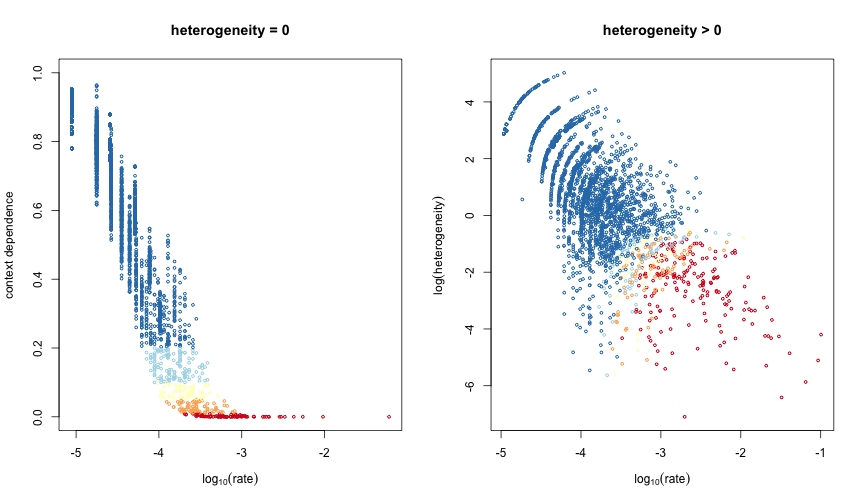Here are the words with the smallest p-values (strongest evidence of neutrality):

``````print(usage %>% group_by(word)
%>% summarize(chisq = sum(chisq_hetero))
%>% mutate(pval = (1 - pchisq(chisq, 1)))
%>% filter(pval < .01)
%>% arrange(pval), n = 50)
``````
``````Source: local data frame [239 x 3]

word     chisq         pval
1         a 152.07364 0.000000e+00
2        an 110.34280 0.000000e+00
3       and 173.01658 0.000000e+00
4        as 129.64024 0.000000e+00
5        be 137.80489 0.000000e+00
6       but  74.75960 0.000000e+00
7        by  89.27938 0.000000e+00
8       for  87.44002 0.000000e+00
9      from 103.26005 0.000000e+00
10     have  90.46017 0.000000e+00
11       in 160.35042 0.000000e+00
12       is  86.16352 0.000000e+00
13       it 121.32879 0.000000e+00
14      not 109.34213 0.000000e+00
15       of 251.93168 0.000000e+00
16       or  89.21787 0.000000e+00
17     that 130.90836 0.000000e+00
18      the 230.46576 0.000000e+00
19     this 127.82706 0.000000e+00
20       to 206.84182 0.000000e+00
21    which 126.63133 0.000000e+00
22     with 108.24181 0.000000e+00
23      all  66.48571 3.330669e-16
24      are  65.30909 6.661338e-16
25       on  64.39968 9.992007e-16
26       if  63.56637 1.554312e-15
27     been  62.54845 2.553513e-15
28     upon  60.25191 8.326673e-15
29      may  60.00842 9.436896e-15
30     such  58.58758 1.942890e-14
31      one  57.45726 3.452794e-14
32       at  56.82302 4.773959e-14
33    those  56.06442 7.016610e-14
34     they  55.74839 8.237855e-14
35    other  54.15432 1.852962e-13
36     than  53.28170 2.889911e-13
37     them  52.90117 3.507195e-13
38    their  52.47549 4.356515e-13
39      has  52.10304 5.265788e-13
40       so  50.34128 1.292078e-12
41 consider  49.31263 2.182587e-12
42    there  47.86499 4.566014e-12
43   publiu  46.84429 7.685630e-12
44      any  45.05366 1.917078e-11
45      its  40.55996 1.906744e-10
46    under  40.21506 2.274876e-10
47       no  38.59517 5.214779e-10
48  subject  38.34817 5.918366e-10
49   object  37.83266 7.708043e-10
50   govern  37.51578 9.067634e-10
..      ...       ...          ...
``````

Here are the words with the largest p-values (weakest evidence of neutrality):

``````print(usage %>% group_by(word)
%>% summarize(chisq = sum(chisq_hetero))
%>% mutate(pval = (1 - pchisq(chisq, 1)))
%>% arrange(chisq), n = 20)
``````
``````Source: local data frame [5,218 x 3]

word     chisq pval
1       court -60.46603    1
2        jury -58.96343    1
3     militia -51.88975    1
4         you -50.05194    1
5       senat -50.04234    1
7       claus -46.15412    1
8      vacanc -42.10553    1
9     impeach -41.25111    1
10   governor -37.03732    1
11       armi -34.53675    1
12     pardon -33.96636    1
13      trial -31.48838    1
14        her -30.72965    1
15      appel -30.11202    1
16     export -29.79590    1
17     presid -29.76895    1
19      amend -28.11362    1
20 manufactur -28.01013    1
..        ...       ...  ...
``````

## Filtering non-distinctive words

We want to filter out the words with similar usages for both authors. To test for the “distinctiveness” of a word, we perform a likelihood ratio test. The null (pooled) model uses the same rate and heterogeneity for both authors; the alternative model uses author-specific rates and heterogeneities.

``````x <- dtm[fed\$author %in% author,]
n <- rowSums(x)
offset <- log(n)
usage_pool <-
do(data_frame(word) %>% group_by(word), { # for each word
y <- x[, .\$word]
fit <- nbinom_fit(y, n) # fit a joint model
as.data.frame(fit)
})

# computed
usage_pool <-
usage_pool %>%
left_join(on = "word",
usage %>% group_by(word)
%>% summarize(chisq_hetero_tot = sum(chisq_hetero),
pval_hetero_tot = 1 - pchisq(chisq_hetero_tot, 1),
deviance_unpool = sum(deviance))) %>%
ungroup()

usage_pool <-
usage_pool %>% mutate(chisq_diff = deviance - deviance_unpool,
pval_diff = 1 - pchisq(chisq_diff, df=2))
``````

The words that discriminate are those where the rates or the heterogeneities differ between the two authors:

``````usage_h <- usage %>% filter(author == "HAMILTON")
usage_m <- usage %>% filter(author == "MADISON")

feature <-
(usage_pool %>% select(word, pval_hetero = pval_hetero_tot, pval_diff)
%>% left_join(on = "word",
usage_h %>% select(word,
rate_h = rate,
hetero_h = heterogeneity))
%>% left_join(on = "word",
usage_m %>% select(word,
rate_m = rate,
hetero_m = heterogeneity)))

par(mfrow=c(1,2))
palette(brewer.pal(5, "RdYlBu"))

with(feature, {
plot(log(rate_h), log(rate_m), cex=0.5,
col=cut(pval_diff,
breaks=c(0, .01, .05, .10, .20, 1),
include.lowest=TRUE))

plot(log(1 + hetero_h), log10(1 + hetero_m), cex=0.5,
col=cut(pval_diff,
breaks=c(0, .01, .05, .10, .20, 1),
include.lowest=TRUE))
})
``````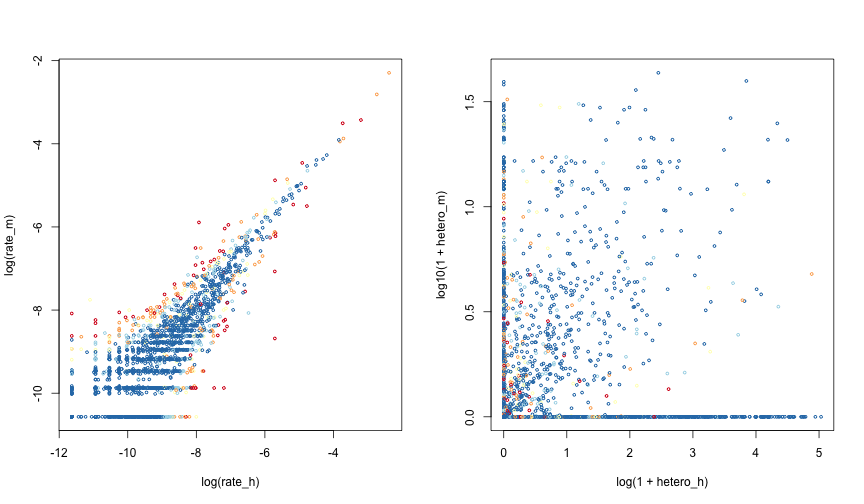After filtering context-sensitive and non-discriminating words, we are left with the following list:

``````print(n=50, feature %>% filter(pval_hetero < .01 & pval_diff < .01)
%>% arrange(pval_diff))
``````
``````Source: local data frame [32 x 7]

word  pval_hetero    pval_diff       rate_h     hetero_h       rate_m
1      upon 8.326673e-15 0.000000e+00 0.0033133998 8.382137e-21 1.694296e-04
2     there 4.566014e-12 1.053376e-09 0.0033090904 5.903965e-02 8.492976e-04
3        on 9.992007e-16 3.785444e-09 0.0033327183 8.932215e-02 7.610774e-03
4        to 0.000000e+00 1.022500e-06 0.0408061102 7.780331e-03 3.243226e-02
5      form 8.785136e-06 1.182165e-06 0.0007679526 5.847613e-02 2.383885e-03
6    intend 4.931379e-03 1.258969e-05 0.0003543743 2.502349e-17 0.000000e+00
7        by 0.000000e+00 5.528195e-05 0.0073734663 6.725293e-02 1.157114e-02
8       and 0.000000e+00 1.773680e-04 0.0239868733 1.325488e-02 3.000255e-02
9     thing 1.262355e-04 1.951483e-04 0.0008213499 6.466986e-02 2.262149e-04
10 circumst 3.690675e-05 5.797961e-04 0.0007796235 1.969892e-21 2.662713e-04
11      men 5.317703e-03 6.977892e-04 0.0013921155 3.204345e-01 5.248330e-04
12   latter 1.535015e-07 7.601439e-04 0.0006644518 6.719843e-19 1.391025e-03
13  readili 6.340043e-03 8.254676e-04 0.0002126246 9.709011e-23 0.000000e+00
14       at 4.773959e-14 8.530972e-04 0.0033784674 1.286970e-01 1.981778e-03
15    fulli 3.471964e-03 1.123578e-03 0.0001328904 4.501896e-21 4.890101e-04
16    would 2.352739e-08 1.672263e-03 0.0084364760 2.885532e-01 4.092781e-03
17    among 6.246744e-03 1.945108e-03 0.0004003216 5.006066e-01 1.048088e-03
18   dispos 2.426478e-03 3.085385e-03 0.0003277962 2.387273e-17 5.147475e-05
19       if 1.554312e-15 3.480529e-03 0.0033771613 9.128662e-02 2.136202e-03
20    those 7.016610e-14 3.694453e-03 0.0028806831 9.128218e-03 1.915001e-03
21   former 9.956616e-06 3.759032e-03 0.0005669989 2.578852e-17 1.131653e-03
22     sens 3.817521e-03 4.372116e-03 0.0006274611 1.222555e-01 1.823271e-04
23   within 4.571486e-04 4.852378e-03 0.0004163898 2.578855e-17 8.949639e-04
24     both 9.369488e-04 5.407189e-03 0.0005104098 4.232261e-01 1.080970e-03
25     also 4.908850e-03 5.587565e-03 0.0003100775 2.180847e-17 7.739099e-04
26       an 0.000000e+00 6.269701e-03 0.0056720418 1.652742e-02 4.246667e-03
27  conduct 9.907637e-04 6.658618e-03 0.0006390427 5.754712e-02 2.340695e-04
28     this 0.000000e+00 6.689284e-03 0.0081578391 4.388663e-03 6.380935e-03
29   happen 1.563822e-03 7.179605e-03 0.0007449865 1.692347e-01 2.584584e-04
30    sever 8.688323e-05 7.467804e-03 0.0007353267 2.414593e-27 1.378155e-03
31   strong 5.401015e-03 8.064146e-03 0.0002923588 1.710871e-20 5.147475e-05
32   observ 7.741428e-06 9.979448e-03 0.0008239203 3.793978e-21 3.991336e-04
Variables not shown: hetero_m (dbl)
``````

## Prior elicitation

We use the neutral words (not just the feature words), to elicit a prior for the word-specific parameters

``````feature <-
feature %>% mutate(s = rate_h + rate_m,
t = ifelse(s == 0, NA, rate_h / s),
z_h = log(1 + hetero_h), # MW use log(1 + rate * hetero)
z_m = log(1 + hetero_m),
x = z_h + z_m,
y = ifelse(x == 0, NA, z_h / x))

par(mfrow=c(1,1))
with(feature %>% filter(pval_hetero < .01), {
plot(sqrt(1000 * s), t, cex=0.5, xlim=c(0, 6))
})

ntot <- sum(dtm[fed\$author %in% c("HAMILTON", "MADISON"),])
s <- seq(.2/1000, 6^2 / 1000, len=200)
lines(sqrt(1000 * s), 0.5 + 2 * sqrt(0.5 * (1 - 0.5) / (ntot * s)))
lines(sqrt(1000 * s), 0.5 - 2 * sqrt(0.5 * (1 - 0.5) / (ntot * s)))
lines(sqrt(1000 * s), 0.55 + 2 * sqrt(0.55 * (1 - 0.55) / (ntot * s)),
lty=2)
lines(sqrt(1000 * s), 0.45 - 2 * sqrt(0.45 * (1 - 0.45) / (ntot * s)),
lty=2)
``````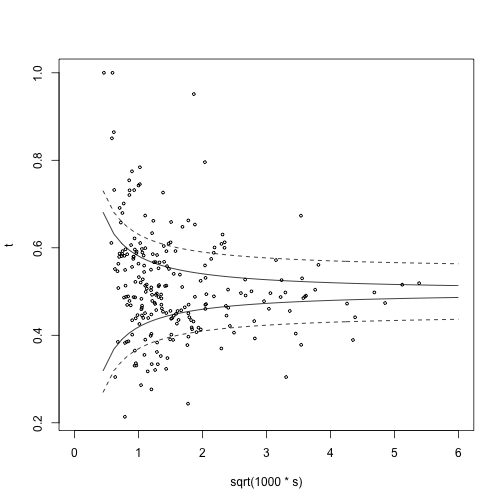Here are the analogues of Mosteller and Wallace's preferred priors:

``````# s = rate_h + rate_m,
# t = ifelse(s == 0, NA, rate_h / s),

palette(brewer.pal(6, "Set1"))
with(feature %>% filter(pval_hetero < .01), {
hist(t, prob=TRUE, 20, col="gray", border="white")
u <- seq(0, 1, len=100)
lines(u, dbeta(u, 10, 10), col=2)
})
``````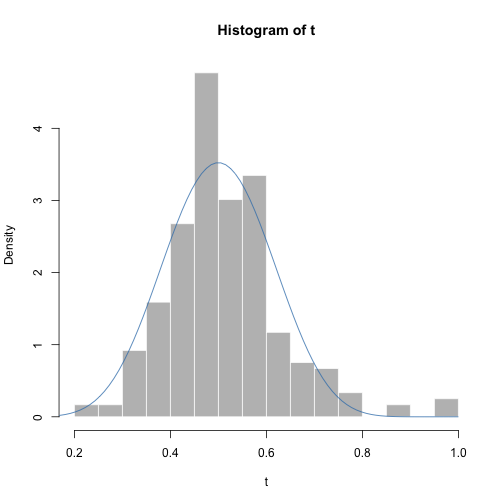``````# y = ifelse(x == 0, NA, z_h / x))

palette(brewer.pal(6, "Set1"))
with(feature %>% filter(pval_hetero < .01), {
hist(y, prob=TRUE, 20, col="gray", border="white")
u <- seq(0, 1, len=100)
lines(u, dbeta(u, 1.2, 1.2), col=2)
})
``````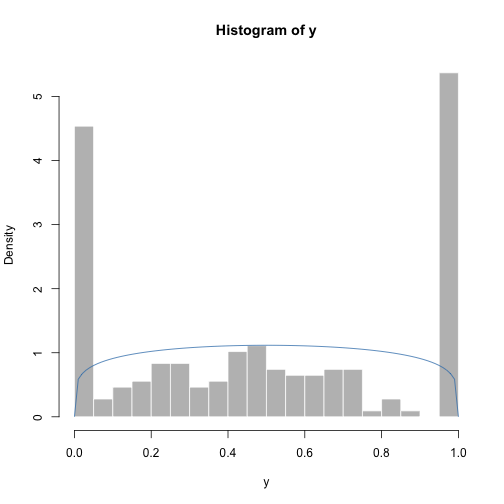``````# z_h = log(1 + hetero_h)
# z_m = log(1 + hetero_m)
# x = z_h + z_m

palette(brewer.pal(6, "Set1"))
with(feature %>% filter(pval_hetero < .01), {
# from https://en.wikipedia.org/wiki/Gamma_distribution#Maximum_likelihood_estimation
s <- log(mean(x[x > 0])) - mean(log(x[x > 0]))
shape <- (3 - s + sqrt((s - 3)^2 + 24 * s)) / (12 * s)
m <- mean(x)
scale <- m / shape
hist(x, prob=TRUE, 20,
main=paste0("mean = ", round(m, 2), ", shape = ", round(shape, 2)),
col="gray", border="white")
u <- seq(0, 2, len=100)
lines(u, dgamma(u, shape=shape, scale=scale), col=2)
})
``````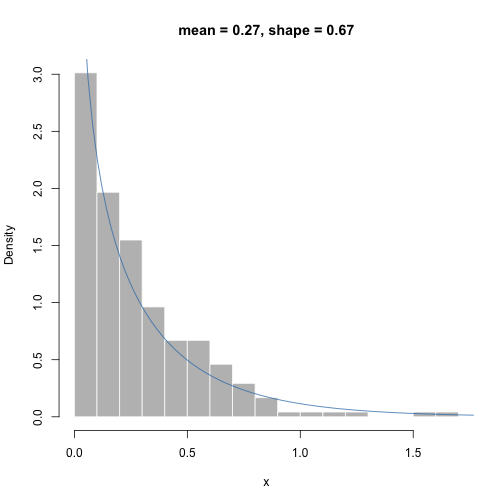After determining the prior, Mosteller and Wallace re-fit the model parameters for each author. Unfortunately, I didn't have time to implement this step. In the remainder of the analysis, we will use the original estimates, note the posterior modes.

## Evaluation

Now, we are ready to evaluate the log odds, the difference in log probabilities between Hamilton and Madison, under the two fitted models.

``````log_odds <-
with(feature %>% filter(pval_hetero < .01 & pval_diff < .01
& rate_h > 0 & rate_m > 0), {
n <- rowSums(dtm)
y <- dtm[,word]
log_odds <- matrix(0, nrow(y), ncol(y))
colnames(log_odds) <- word
rownames(log_odds) <- fed\$paper_id

for (j in seq_len(ncol(y))) {
# Hamilton
mu_h = n * rate_h[j]
size_h <- 1/hetero_h[j]
if (is.finite(size_h)) {
lp_h <- dnbinom(y[,j], mu=mu_h, size=size_h, log=TRUE)
} else {
lp_h <- dpois(y[,j], mu_h, log=TRUE)
}

mu_m = n * rate_m[j]
size_m <- 1/hetero_m[j]
if (is.finite(size_m)) {
lp_m <- dnbinom(y[,j], mu=mu_m, size=size_m, log=TRUE)
} else {
lp_m <- dpois(y[,j], mu_m, log=TRUE)
}

log_odds[,j] <- lp_h - lp_m
}
log_odds
})
``````

Here are the log odds of Hamilton authorship for all 85 papers.

``````fed %>% select(paper_id, author) %>% mutate(log_odds = rowSums(log_odds))
``````
``````   paper_id               author    log_odds
1         1             HAMILTON  16.4797642
2         2                  JAY -15.8383145
3         3                  JAY  -0.5422449
4         4                  JAY  -4.4912495
5         5                  JAY   0.7456437
6         6             HAMILTON  15.0944817
7         7             HAMILTON  30.1168259
8         8             HAMILTON  14.6402354
9         9             HAMILTON  12.9441877
11       11             HAMILTON  32.2205454
12       12             HAMILTON  22.7261210
13       13             HAMILTON  19.9354743
15       15             HAMILTON  50.3237556
16       16             HAMILTON  36.3816007
17       17             HAMILTON  23.0747751
18       18 HAMILTON AND MADISON -19.7301315
19       19 HAMILTON AND MADISON -26.6078391
20       20 HAMILTON AND MADISON -10.1594784
21       21             HAMILTON  16.2466009
22       22             HAMILTON  41.2528649
23       23             HAMILTON  23.0683465
24       24             HAMILTON  22.3036606
25       25             HAMILTON  16.0257315
26       26             HAMILTON  31.8900006
27       27             HAMILTON  25.2222312
28       28             HAMILTON  26.5109723
29       29             HAMILTON  47.3356987
30       30             HAMILTON  21.4429595
31       31             HAMILTON  25.4966420
32       32             HAMILTON  14.7587032
33       33             HAMILTON  16.0600232
34       34             HAMILTON  26.4646869
35       35             HAMILTON  31.8856120
36       36             HAMILTON  39.0888761
49       49  HAMILTON OR MADISON  -7.7306485
50       50  HAMILTON OR MADISON  -7.7821363
51       51  HAMILTON OR MADISON -26.8142277
52       52  HAMILTON OR MADISON -13.3552654
53       53  HAMILTON OR MADISON -19.3127723
54       54  HAMILTON OR MADISON -12.7966779
55       55  HAMILTON OR MADISON   1.8015920
56       56  HAMILTON OR MADISON -24.3258065
57       57  HAMILTON OR MADISON -10.5319213
58       58  HAMILTON OR MADISON -16.3282432
59       59             HAMILTON  28.5427436
60       60             HAMILTON  33.7538588
61       61             HAMILTON  26.2893422
62       62  HAMILTON OR MADISON -21.9147956
63       63  HAMILTON OR MADISON -11.3698113
64       64                  JAY -10.2755475
65       65             HAMILTON  28.2505817
66       66             HAMILTON  27.7044486
67       67             HAMILTON  21.8855079
68       68             HAMILTON  15.1564381
69       69             HAMILTON  17.7331915
70       70             HAMILTON  32.2904911
71       71             HAMILTON  20.2022523
72       72             HAMILTON  32.6928452
73       73             HAMILTON  30.1328744
74       74             HAMILTON  18.8967062
75       75             HAMILTON  22.9016649
76       76             HAMILTON  35.1282946
77       77             HAMILTON  30.4134857
78       78             HAMILTON  30.7213625
79       79             HAMILTON   9.5350036
80       80             HAMILTON  16.3421459
81       81             HAMILTON  36.2759476
82       82             HAMILTON  16.7660266
83       83             HAMILTON  51.3096150
84       84             HAMILTON  23.1671353
85       85             HAMILTON  21.3656825
``````

## Discussion

Our analysis is incomplete, because we did not use the priors for the word parameters in computing the authorship log odds. We also failed to investigate the strength and effect of dependence between the word counts. In light of this, our results are only preliminary.

For most of the papers, our analysis agrees with Mosteller and Wallace. We have very strong evidence of Madison authorship for most of the papers. For Paper No. 55, we found weak evidence of Hamilton authorship; Mosteller and Wallace found weak evidence of Madison authorship for this paper.

One notable difference between our analyis and Mosteller and Wallace's is that we do the feature selection completely automatically. This allows our approach to easily adapt to other datasets and applications, but it is also a potential weakness, because our tests for neutrality might be less reliable than Mosteller and Wallace's subjective judgments.

## Session information

``````sessionInfo()
``````
``````R version 3.2.3 (2015-12-10)
Platform: x86_64-apple-darwin13.4.0 (64-bit)
Running under: OS X 10.10.5 (Yosemite)

locale:
 en_US.UTF-8/en_US.UTF-8/en_US.UTF-8/C/en_US.UTF-8/en_US.UTF-8

attached base packages:
 methods   stats     graphics  grDevices utils     datasets  base

other attached packages:
 tm_0.6-2           SnowballC_0.5.1    openNLP_0.2-5      NLP_0.1-8
 Matrix_1.2-3       jsonlite_0.9.16    dplyr_0.4.1        RColorBrewer_1.1-2
 knitr_1.9

loaded via a namespace (and not attached):
 Rcpp_0.11.5         codetools_0.2-14    lattice_0.20-33
 digest_0.6.8        assertthat_0.1      slam_0.1-32
 grid_3.2.3          DBI_0.3.1           formatR_1.1
 magrittr_1.5        evaluate_0.6        lazyeval_0.1.10
 openNLPdata_1.5.3-2 tools_3.2.3         stringr_0.6.2
 parallel_3.2.3      rJava_0.9-8
``````